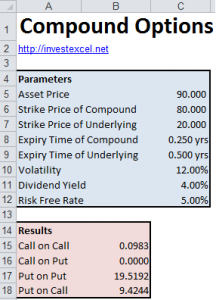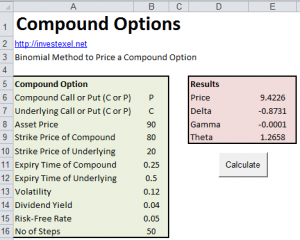# Compound Options – Introduction and Pricing Spreadsheet

This article introduces compound options, and provides a pricing spreadsheet. Companies often use compound options when tendering for a contract; the option hedges against the risk of winning the contract. They are also used in foreign currency and fixed income markets, when risk protection may or may not be required. They allow the holder to pay a lower initial premium.

A compound option is an option on an option – in other words, an option to trade another option. A premium is first paid for the compound option.  At the expiry, the holder decides whether to exercise the compound option. If it is exercised, the holder receives an option on the underlying but has to pay a second premium. If both options are exercised, then the compound option will be more expensive than an vanilla option on the underlying.

Two sets of parameters, one each for the underlying and compound option, are required.  As such, the combined volatility (that is, the volatility of volatility) of both options significantly affects their price.

Four combinations can be defined.

• Call on Call. These payout max{C(S,T1) – X1,0} and have a price of
• Call on Put. These payout of max{P(S,T1) – X1, 0} and have a price of
• Put on Call. These payout of max{X1 – C(S,T1) ,0} and have a price of
• Put on Put. These payout of max{X1– P(S,T1) ,0} and have a price of
where
• T1 and X1 are the first exercise date and strike price
• T2 and X2 are the second exercise date and strike price
• S is the current stock price
• r si the interest rate
• q is the dividend rate
• σ is the volatility
• S* is the stock price at T1 for which the option price is X1. It is calculated iteratively
• M(a, b, ρ) is the bivariate cumulative distribution function

Rubinstein (1991) and Geske (1979) derived these equations. They assume constant volatility, and hence underestimate the price.

Other options have many of the characteristics of compound options.

• Chooser options let the holder choose whether the option is a call or a put after a fixed time.
• Installment options let the holder pay a premium in installments. After each payment, the holder can exercise or terminate the option.
• Extendible options let the holder trade the option, or extend its maturity.

## Pricing Compound Options in ExcelThis Excel spreadsheet prices compound options with the equations given by Rubinstein (1991) and Geske (1979). It is coded in VBA and uses an approximation for the bivariate cumulative probability distribution function.

## Pricing Compound Options with a Binomial Tree

This Excel spreadsheet prices compound options with a Cox-Ross-Rubinstein binomial tree, and also calculates the Greeks (Delta, Gamma and Theta). The routine is coded in VBA (leave a comment if you want the password).Both this and the earlier spreadsheet gives similar results. Binomial option pricing is described in detail here.### 11 thoughts on “Compound Options – Introduction and Pricing Spreadsheet”

1. Very resourceful information. May I have the password please? It would be really helpful for me to understand further. Thank you. =]

• Sure – which specific spreadsheet do you want?

2. Hi
Have you programmed the bivariate cumulative distribution function, or used some other method?

3. Hi, this is a rather informative guide. Would it be possible to have the password for the Compound Options Pricing with Binomial Tree? Thanks! 🙂

4. Hi, is it possible to have CRR method to price barrier options
THanks

5. What excel version are you using? I am using 2007 version and the macros are not working. Thanks!

• Excel 2010 on Windows 7

6. The inputs:
Is the time to maturity specified in years if the risk-free rate and volatility figures are in years?
On the compound option model, if the compound dividend has a, say, 1 year life and entitles the holder to acquire the underlying option which then has a 1 year life from the date of acquisition, are the expiry times 1 & 1 or 1 & 2
Is the risk free rate a decimal or a percentage? i.e. 0.1 = 10% not 0.1=0.1% (interest rates are so low at present, it is a bit difficult to guess from the examples)
Thanks

• >Is the time to maturity specified in years if the risk-free rate and volatility figures are in years?

Yes

>Is the risk free rate a decimal or a percentage?

On the spreadsheet, enter a percentage (i.e. a number between 0 and 100)